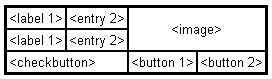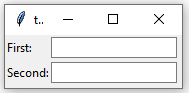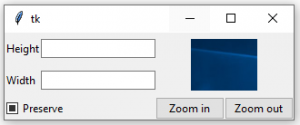# Python | grid() method in Tkinter

• Difficulty Level : Basic
• Last Updated : 21 Apr, 2022

The Grid geometry manager puts the widgets in a 2-dimensional table. The master widget is split into a number of rows and columns, and each “cell” in the resulting table can hold a widget. The grid manager is the most flexible of the geometry managers in Tkinter. If you don’t want to learn how and when to use all three managers, you should at least make sure to learn this one. Consider the following example –Creating this layout using the pack manager is possible, but it takes a number of extra frame widgets, and a lot of work to make things look good. If you use the grid manager instead, you only need one call per widget to get everything laid out properly. Using the grid manager is easy. Just create the widgets, and use the grid method to tell the manager in which row and column to place them. You don’t have to specify the size of the grid beforehand; the manager automatically determines that from the widgets in it.

Code #1:

## Python3

 `# import tkinter module` `from` `tkinter ``import` `*` `from` `tkinter.ttk ``import` `*`   `# creating main tkinter window/toplevel` `master ``=` `Tk()`   `# this will create a label widget` `l1 ``=` `Label(master, text ``=` `"First:")` `l2 ``=` `Label(master, text ``=` `"Second:")`   `# grid method to arrange labels in respective` `# rows and columns as specified` `l1.grid(row ``=` `0``, column ``=` `0``, sticky ``=` `W, pady ``=` `2``)` `l2.grid(row ``=` `1``, column ``=` `0``, sticky ``=` `W, pady ``=` `2``)`   `# entry widgets, used to take entry from user` `e1 ``=` `Entry(master)` `e2 ``=` `Entry(master)`   `# this will arrange entry widgets` `e1.grid(row ``=` `0``, column ``=` `1``, pady ``=` `2``)` `e2.grid(row ``=` `1``, column ``=` `1``, pady ``=` `2``)`   `# infinite loop which can be terminated by keyboard` `# or mouse interrupt` `mainloop()`

Output:Code #2: Creating the layout which is shown above.

## Python3

 `# import tkinter module` `from` `tkinter ``import` `*` `from` `tkinter.ttk ``import` `*`   `# creating main tkinter window/toplevel` `master ``=` `Tk()`   `# this will create a label widget` `l1 ``=` `Label(master, text ``=` `"Height")` `l2 ``=` `Label(master, text ``=` `"Width")`   `# grid method to arrange labels in respective` `# rows and columns as specified` `l1.grid(row ``=` `0``, column ``=` `0``, sticky ``=` `W, pady ``=` `2``)` `l2.grid(row ``=` `1``, column ``=` `0``, sticky ``=` `W, pady ``=` `2``)`   `# entry widgets, used to take entry from user` `e1 ``=` `Entry(master)` `e2 ``=` `Entry(master)`   `# this will arrange entry widgets` `e1.grid(row ``=` `0``, column ``=` `1``, pady ``=` `2``)` `e2.grid(row ``=` `1``, column ``=` `1``, pady ``=` `2``)`   `# checkbutton widget` `c1 ``=` `Checkbutton(master, text ``=` `"Preserve")` `c1.grid(row ``=` `2``, column ``=` `0``, sticky ``=` `W, columnspan ``=` `2``)`   `# adding image (remember image should be PNG and not JPG)` `img ``=` `PhotoImage(``file` `=` `r"C:\Users\Admin\Pictures\capture1.png")` `img1 ``=` `img.subsample(``2``, ``2``)`   `# setting image with the help of label` `Label(master, image ``=` `img1).grid(row ``=` `0``, column ``=` `2``,` `       ``columnspan ``=` `2``, rowspan ``=` `2``, padx ``=` `5``, pady ``=` `5``)`   `# button widget` `b1 ``=` `Button(master, text ``=` `"Zoom ``in``")` `b2 ``=` `Button(master, text ``=` `"Zoom out")`   `# arranging button widgets` `b1.grid(row ``=` `2``, column ``=` `2``, sticky ``=` `E)` `b2.grid(row ``=` `2``, column ``=` `3``, sticky ``=` `E)`   `# infinite loop which can be terminated ` `# by keyboard or mouse interrupt` `mainloop()`

Output:Warning: Never mix grid() and pack() in the same master window.

My Personal Notes arrow_drop_up
Recommended Articles
Page :